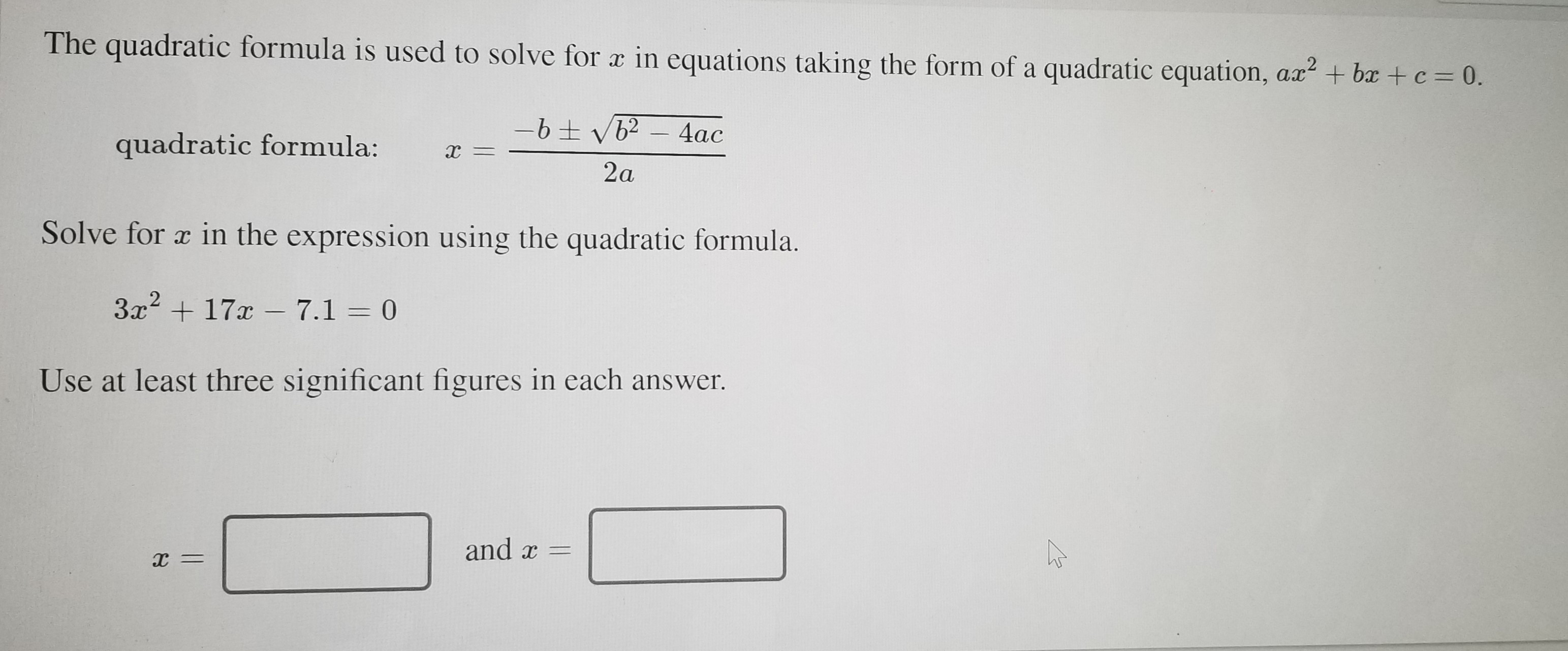# The quadratic formula is used to solve for x in equations taking the form of a quadratic equation, ax? + bx + c = 0. 4ac quadratic formula: х — 2a Solve for a in the expression using the quadratic formula. 3x + 17x – 7.1 = 0 Use at least three significant figures in each answer. and x = х —

Questionhelp_outlineImage TranscriptioncloseThe quadratic formula is used to solve for x in equations taking the form of a quadratic equation, ax? + bx + c = 0. 4ac quadratic formula: х — 2a Solve for a in the expression using the quadratic formula. 3x + 17x – 7.1 = 0 Use at least three significant figures in each answer. and x = х — fullscreen

1 Rating

### Want to see this answer and more?

Experts are waiting 24/7 to provide step-by-step solutions in as fast as 30 minutes!*

*Response times may vary by subject and question complexity. Median response time is 34 minutes for paid subscribers and may be longer for promotional offers.
Tagged in
Math
Algebra

### Other